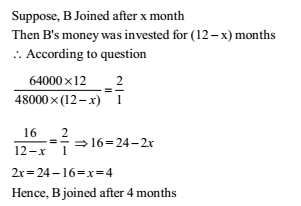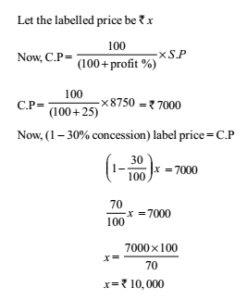# RRB ALP 2018 Practice Test Papers | Arithmetic Questions (Day-39)

Dear Aspirants, Here we have given the Important RRB ALP & Technicians Exam 2018 Practice Test Papers. Candidates those who are preparing for RRB ALP 2018 can practice these Arithmetic Questions to get more confidence to Crack RRB 2018 Examination.

[WpProQuiz 2147]

Click “Start Quiz” to attend these Questions and view Explanation

1. 3 daily wage workers A, B and C are distributed Rs. 178 in such a way that A gets Rs. 4 less than C, B gets Rs.  15 more than A and C gets Rs.  11 less than B. What is the ratio of their shares?

(a) 53: 68: 57

(b) 57: 53: 68

(c) 50: 51: 52

(d) 53: 56: 68

1. What is the height of a cylinder that has the same volume and radius as a sphere of diameter 12 cm?

(a) 8 cm

(b) 7 cm

(c) 10 cm

(d) 9 cm

1. The average of 30 numbers is 40 and that of other 40 numbers is 30. The average of all the numbers is

(a) 34.5

(b) 34 2/ 7

(c) 35

(d) 34

1. The length and breadth of a rectangle are doubled. Percentage increase in area is

(a) 400%

(b) 150%

(c) 200%

(d) 300%

1. In the annual examination Mahuya got 10% less marks than Supriyo in Mathematics. Mahuya got 81 marks. The marks of Supriyo are

(a) 89

(b) 90

(c) 87

(d) 88

1. A invests Rs. 64,000 in a business. After few months B joined him with Rs. 48,000. At the end of year, the total profit was divided between them in the ratio 2: 1. After how many months did B join?

(a) 7

(b) 8

(c) 4

(d) 6

1. A got 30% concession on the label price of an article sold for Rs. 8,750 with 25% profit on the price he bought. The label price was

(a) 10,000

(b) 13,000

(c) 16,000

(d) 12,000

1. The least number that should be added to 2055 so that the sum is exactly divisible by 27 is:

(a) 24

(b) 27

(c) 31

(d) 28

1. What least value must be assigned to ‘*’ so that the numbers 451*603 is exactly divisible by 9?

(a) 7

(b) 8

(c) 5

(d) 9

1. A basket contains 300 mangoes. 75 mangoes were distributed among some students. Find the percentage of mangoes left in the basket

(a) 70%

(b) 72%

(c) 76%

(d) 75%

A = C – 4 …….(1)

B = A + 15 …….(2)

C = B – 15 …….(3)

From (1) and (3)

A = B – 11 – 4

A = B – 15

A: B: C

B – 15: B: B – 11

B – 15 + B + B – 11 = 178

3 B = 178 + 26 = 204 => B = 68

A = 53, C = 57

Volume of cylinder = volume of sphere (Given)

pi r2 h =4/3 pi r3

h=4/3 r

4/3×6 = 8 cm

Sum of 30 numbers = 30×40 = 1200 Sum of 40 numbers = 40 × 30 = 1200

Average of 70 numbers = (1200 +1200)/7 = 2400/7 = 34 2/ 7

A = lb

A’ = (2l)(2b) = 4lb = 4A

% Change = 4A-A/A x100=300%

Marks of Supriyo = x marks

Accoding to question Mahuya marks = Supriyo marks – 10% of Supriyo marks

81 = x – 10% of x

81 = 9/10 x => x = 90 marks

1. Answer: (c)2. Answer: (a)Number has to be less than 27.

Let the number be x. On Dividing 2055 by 27, we get remainder as 3 Now, 3 + x = 27

x = 24

To divide 451 * 603 by 9

(4 + 5 + 1 + * + 6 + 0 + 3) = (19 + *)

(19 + *) must be multiple of 9

19 + * = 27 * = 8

Total mango = 300

Distribution = 75

Distributed% = 75/300 ×100 = 25%

Percentage left in the basket = 75%

RRB ALP 2018 Practice Test Papers | Arithmetic Questions (Day-1)

RRB ALP 2018 Practice Test Papers | Arithmetic Questions (Day-2)

RRB ALP 2018 Practice Test Papers | Arithmetic Questions (Day-3)

RRB ALP 2018 Practice Test Papers | Arithmetic Questions (Day-4)

RRB ALP 2018 Practice Test Papers | Arithmetic Questions (Day-5)

RRB ALP 2018 Practice Test Papers | Arithmetic Questions (Day-6)

RRB ALP 2018 Practice Test Papers | Arithmetic Questions (Day-7)

RRB ALP 2018 Practice Test Papers | Arithmetic Questions (Day-8)

RRB ALP 2018 Practice Test Papers | Arithmetic Questions (Day-9)

RRB ALP 2018 Practice Test Papers | Arithmetic Questions (Day-10)

RRB ALP 2018 Practice Test Papers | Arithmetic Questions (Day-11)

RRB ALP 2018 Practice Test Papers | Arithmetic Questions (Day-12)

RRB ALP 2018 Practice Test Papers | Arithmetic Questions (Day-13)

RRB ALP 2018 Practice Test Papers | Arithmetic Questions (Day-14)

RRB ALP 2018 Practice Test Papers | Arithmetic Questions (Day-15)

RRB ALP 2018 Practice Test Papers | Arithmetic Questions (Day-16)

RRB ALP 2018 Practice Test Papers | Arithmetic Questions (Day-17)

RRB ALP 2018 Practice Test Papers | Arithmetic Questions (Day-18)

RRB ALP 2018 Practice Test Papers | Arithmetic Questions (Day-19)

RRB ALP 2018 Practice Test Papers | Arithmetic Questions (Day-20)

RRB ALP 2018 Practice Test Papers | Arithmetic Questions (Day-21)

RRB ALP 2018 Practice Test Papers | Arithmetic Questions (Day-22)

RRB ALP 2018 Practice Test Papers | Arithmetic Questions (Day-23)

RRB ALP 2018 Practice Test Papers | Arithmetic Questions (Day-24)

RRB ALP 2018 Practice Test Papers | Arithmetic Questions (Day-25)

RRB ALP 2018 Practice Test Papers | Arithmetic Questions (Day-26)

RRB ALP 2018 Practice Test Papers | Arithmetic Questions (Day-27)

RRB ALP 2018 Practice Test Papers | Arithmetic Questions (Day-28)

RRB ALP 2018 Practice Test Papers | Arithmetic Questions (Day-29)

RRB ALP 2018 Practice Test Papers | Arithmetic Questions (Day-30)

RRB ALP 2018 Practice Test Papers | Arithmetic Questions (Day-31)

RRB ALP 2018 Practice Test Papers | Arithmetic Questions (Day-32)

RRB ALP 2018 Practice Test Papers | Arithmetic Questions (Day-33)

RRB ALP 2018 Practice Test Papers | Arithmetic Questions (Day-34)

RRB ALP 2018 Practice Test Papers | Arithmetic Questions (Day-35)

RRB ALP 2018 Practice Test Papers | Arithmetic Questions (Day-36)

RRB ALP 2018 Practice Test Papers | Arithmetic Questions (Day-37)

RRB ALP 2018 Practice Test Papers | Arithmetic Questions (Day-38)